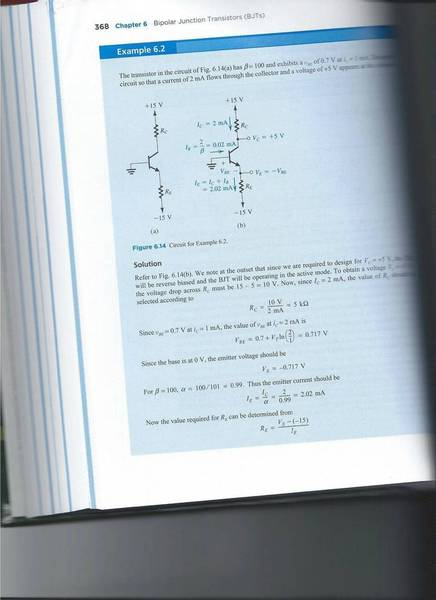# Where would this transistor fail?

tamtam402
I succesfully did this example from my book without checking the solution, but I came up with a question that I cannot answer on my own. What would happen if Re was set lower?

The base is grounded and Vbe is independant of the current Ie, so by choosing a resistance smaller than 7070ohm for Re, it seems like the equation Ie = Ib + Ic wouldn't hold anymore, which is impossible. So, what would happen if I were to set a Re too small?

Basically, I have a problem where the book says the emitter current should be 2.02mA. Yes, that's what I calculated, but what would happen if I were to set a value for Re that makes would give a 3mA current, for example?

Since the scan didn't work properly (the book is huge and I couldn't make the whole page fit flat), here's the first paragraph:

The transistor in the circuit of Fig. 6.14(a) has B=100 and exhibits a Vbe of 0.7V at Ic=1mA. Design the circuit so that a current of 2Ma flows through the collector and a voltage of +5V appears at the collector.

Edit: I didn't scan the second page. It's simply the last equation of the first page with the values replaced for Ve (-0.717V) and Ie (2.02mA).These formulas only apply as long as the transistor is adequately biased and operating linearly.

The emitter resistor in this case would be about 7.15 K. (15 V- 0.7V) /0.002 A = 14.3 V / 0.002 A or about 7.15 K.

If you wanted 3 mA then the emitter resistor would be (15 V- 0.7V) /0.003 A or 4.77 K.

This depends on there being sufficient current available from the collector circuit, so you need to make this a part of a complete design. Changing the emitter resistor must be accompanied by a redesign of the collector circuit if the output voltage of 5 volts is to be retained.

In this case if the emitter current was to be 3 mA then the collector resistor would have to be about 10 V / 0.003 A or 3.33 K.
Notice that the actual collector current would be 3 mA - 0.01 * 3 mA or 2.97 mA, but this makes little difference to the final calculation and you would still use a 3.3 K resistor.

tamtam402
Yes I understand that the resistance must be about 7k, I used the same formula you used. I was simply wondering what would happen if the resistance was set lower, thus forcing a Ie to be bigger than excepted. I just answered my own question though, and I'll explain it just in case anyone else was wondering.

In my first post I stated that Vb would be unaffected by a change in the current Ie. That's simply not true, because we know Vb = Ve + 0.7V. By changing the resistor to a lower value, the current Ie increases and the voltage Ve will also be higher. The voltage Vbe wouldn't be 0.7V anymore, and the transitor wouldn't be in active (or saturation) mode. No current would flow since it would have to be in cut-off.

Jony130
In my first post I stated that Vb would be unaffected by a change in the current Ie. That's simply not true, because we know Vb = Ve + 0.7V. By changing the resistor to a lower value, the current Ie increases and the voltage Ve will also be higher. The voltage Vbe wouldn't be 0.7V anymore, and the transitor wouldn't be in active (or saturation) mode. No current would flow since it would have to be in cut-off.
No, by changing the Re resistor to a lower value BJT will enter saturation region not cut-off.
Ic_max = Vcc/Rc = 15V/5K = 3mA
So If Ie > 3mA the BJT will enter saturation region.